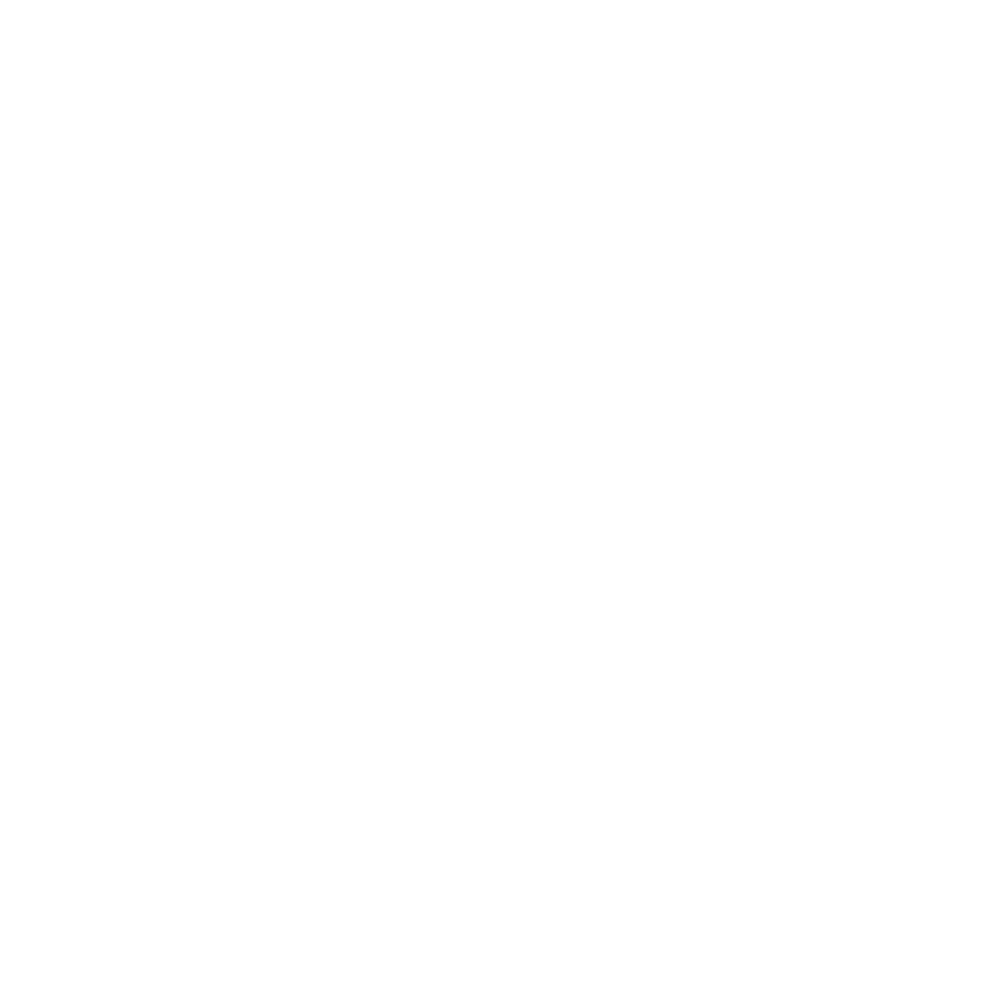# Calibration and Quantitation Training Course

1 Day

## Discover the most common calculation methods used in support of a typical quantitative instrument analytical analysis

This one day course covers the most common methods used in the calculation, calibration and quantitation of data obtained from instrumental analysis. The material is extensively workshop driven, building your understanding of the different methods and when they would be applied. You will gain confidence in working with all types of calculated data.

• Basic proportional calculations and unit conversions
• Methods of standardising calibrations
• Methods for improving data confidence

### Standards

• Preparative challenges
• Standard verification
• Salt correction factor

### Basic Proportional Calculations

• Proportion
• Beer's Law
• Two point linear regression
• Multipoint standard calibration
• Bracketing

### Linear Calibration Lines

• Correlation co-efficient
• Residuals
• Confidence Intervals
• Outliers
• Residual sum of squares
• Use of the origin

### Sample Purity

• Percent area normalisation
• Relative response factor

### Verification of calibration performance

• QCs and check standards
• Surrogates

### Line weighting

• Ordinary least squares regression
• Inverse weighting
• When do we use modified weighting?

### System Suitability

• Placebo and blanks
• Signal to noise
• LOD/LOQ
• Calibration verification
• In sequence checks
• Precision
• Chromatographic peak shape and resolution

This course is relevant to anyone involved in the generation or review of data associated with instrumental analytical chemical techniques such as HPLC, GC, mass spec, molecular and atomic spectroscopy.

Good knowledge of chromatography and associated data.

Understanding HPLC

Operating and understanding GC

GC-MS

LC-MS

Analytical method validation